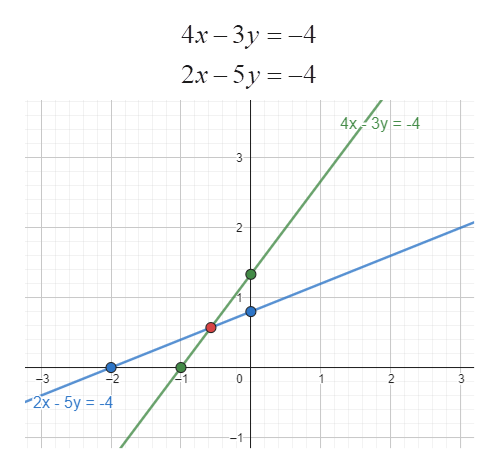# 4x-3y=-4 and 2x-5y=-4. I need to find a solution and solve it graphically

Question
2 views

4x-3y=-4 and 2x-5y=-4. I need to find a solution and solve it graphically

check_circle

Step 1

Given linear equations are:

The lines representing the linear equations are to be plotted and the intersection of the graph gives the solution for the linear equations.

Plotting the graphs using the intercepts:

For the first equation i.e. 4x – 3y = -4:

When x = 0, y = 4/3

When y = 0, x = -1

Hence the intercepts of the line are (0,4/3) and (-1,0)

For the second equation i....help_outlineImage Transcriptionclose4х - Зу %3D — 4 2х - 5у%3 -4 4x, Зу—-4 3 2 -3 0 2 3 2х - 5y %3D -4 -1 от fullscreen

### Want to see the full answer?

See Solution

#### Want to see this answer and more?

Solutions are written by subject experts who are available 24/7. Questions are typically answered within 1 hour.*

See Solution
*Response times may vary by subject and question.
Tagged in

### Equations and In-equations